# Convolutions, Separable Kernels and Gaussian FilterNikan Feb 04, 2020 · 22 mins read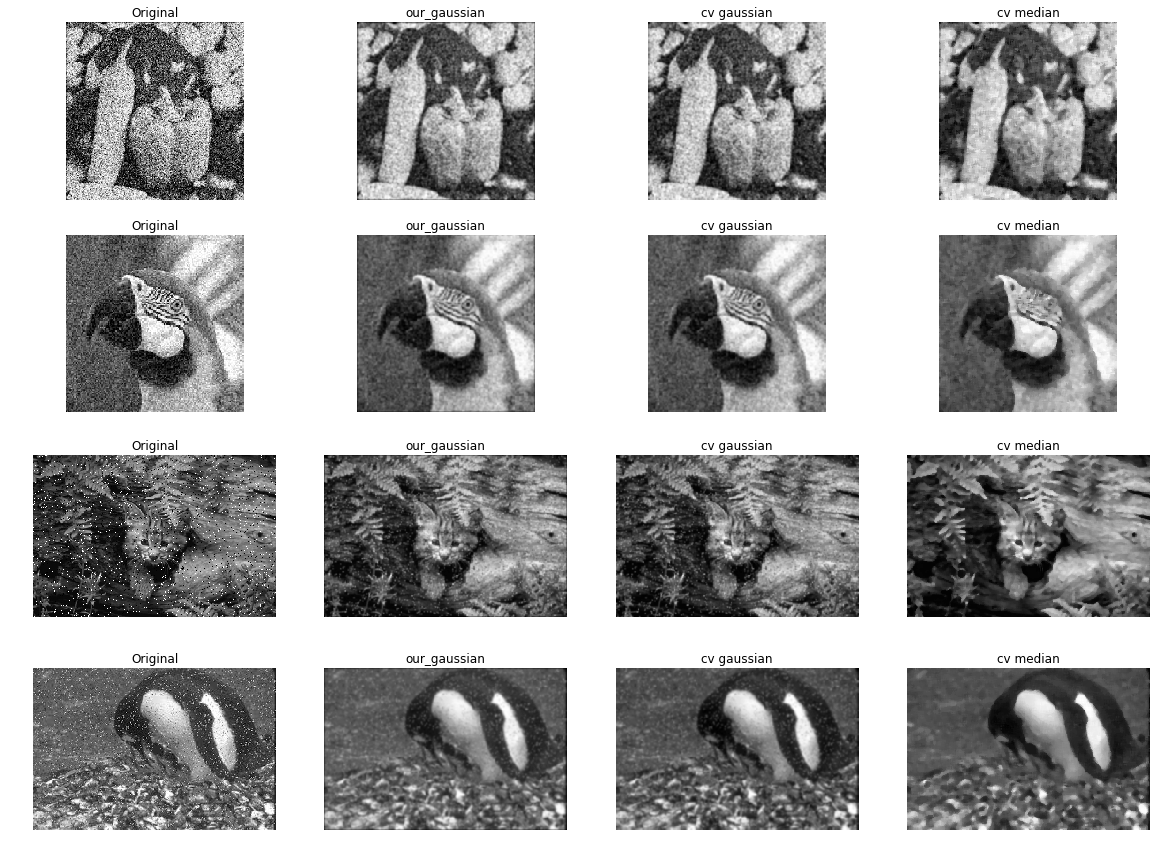# Convolutions, Separable Kernels and Gaussian Filter

This post consists of:

1. Give short answers to below questions:
1. How many zeros are needed to have a same convolution over a 256x256 with a 5x5 filter?
2. What is the requirement of being Separable Kernels?
3. How much speed-up will be up obtained if we use separated kernels of a 31x31 kernel?
2. Write the separated kernels of the below filter and explain what they will do. \begin{bmatrix} -1 & 0 & 1
-2 & 0 & 2
-1 & 0 & 1 \end{bmatrix}
3. Instructions to obtain separated kernels
4. What is their jobs?

5. Calculate below three tasks on paper:
1. Compute the norm of following vectors: \begin{equation} x= \begin{bmatrix} 1+j
2
1-j \end{bmatrix} , y= \begin{bmatrix} 1
-1
1 \end{bmatrix} \end{equation
}
6. Calculate the inner product of x and y. Are they orthonormal?

7. Find degree between two following vectors: \begin{equation} x= \begin{bmatrix} 1
j
1-j \end{bmatrix} , y= \begin{bmatrix} -1
-j
j-1 \end{bmatrix} \end{equation
}

8. Implement functions described in the following tasks:
1. Implement make_gaussian regarding 3.36 formula in reference book. Note that size is same (size*size) and the output filter must be 2D with arbitrary size and std.
2. Implement convolve2d with respect to the given image and kernel. You can use 3.35 formula in reference book.
3. Wrap up median and gaussian built-in functions from cv2 in predefined functions.
4. Discuss the outputs using provided code and indicate the time they consumed.

## 1 Short Answers

1. How many zeros are needed to have a same convolution over a 256x256 with a 5x5 filter?
2. What is the requirement of being Separable Kernels?
3. How much speed-up will be up obtained if we use separated kernels of a 31x31 kernel?

### 1.A

Firstly, We use following formula to calculate p or padding size: \begin{equation} x_n = \lfloor{x_o - f + 2p} \rfloor + 1 \end{equation}

Then we can get number of zeros using this formula: \begin{equation} nz = 4x_n + 4y_n + 4p^2 \end{equation}

import numpy as np
from scipy import ndimage
import matplotlib.pyplot as plt

image_size = 256
image_size_new = image_size
filter_size = 5
p = (image_size_new - image_size -1 + filter_size) // 2
x = np.ones((image_size, image_size))
f = np.ones((filter_size, filter_size))

nz = 4*image_size_new + 4*image_size_new + 4*p**2
print('Number of zeros:', nz)

(260, 260)
Number of zeros from "np.pad" function: 2064
Number of zeros: 2064


### 1.B Separable Kernels

We know the output matrices of the result of multiplying a row and a column matrix is rank 1. So the only requirement of being separable is that the kernel itself must be rank 1. Here is an example:

\begin{equation} x= \begin{bmatrix} 1
1 \end{bmatrix} . y= \begin{bmatrix} 1 & 1 & 1 \end{bmatrix}= \begin{bmatrix} 1 & 1 & 1
1 & 1 & 1 \end{bmatrix} \end{equation
}

print('Rank of aforementioned matrix:',np.linalg.matrix_rank([[1, 1, 1], [1, 1, 1]]))

Rank of aforementioned matrix: 1


### 1.C Speed Up of Separable Kernels

We follow this formula which exists in reference book: \begin{equation} speed = \frac{MNmn}{MNm+MNn} = \frac{mn}{m+n} \end{equation}

f = 31
speed = f*f / (f+f)
print('Speed up {}x'.format(speed))

Speed up 15.5x


## 2 Functionality of Separable Kernels of Kernel so-called Sobel

\begin{bmatrix} -1 & 0 & 1
-2 & 0 & 2
-1 & 0 & 1 \end{bmatrix}

1. Getting Separable Kernels
2. Functionalities

### 2.A Getting Separable Kernels

1. Choose any non-zero random number from the given matrix and call it E
2. Get E’s column as it is and call it V
3. Get E’s row and call it r
4. Calculate omega = r/E, V and omega are the kernels
5. You can get original kernel by calculating w = V * omega.
kernel = np.array(
[[-1, 0, 1],
[-2, 0, 2],
[-1, 0, 1]])
E_index = kernel.nonzero(), kernel.nonzero()
V = kernel[:,E_index].reshape(-1,1)
r = kernel[E_index,:].reshape(1,-1)
omega = r/kernel[E_index]
w = omega * V
print(w)
print(w.all() == kernel.all())

[[-1.  0.  1.]
[-2.  0.  2.]
[-1.  0.  1.]]
True


### 2.B Functionalities

1. First Kernel \begin{bmatrix} -1
-2
-1 \end{bmatrix}
2. Second Kernel \begin{bmatrix} -1
0
1 \end{bmatrix}

#### 2.B.a

\begin{bmatrix} -1
-2
-1 \end{bmatrix}

r = 150
x = np.array([[r/5, r/5 , r],
[r,r/5, r],
[r, r/5 , r/5]
])
fig, ax = plt.subplots(nrows=2, ncols=1, figsize=(5, 8))
ax.imshow(x, cmap='gray')
x_conv = ndimage.convolve(x, [[1,2,1]])
x_conv[x_conv > 255] = 255
ax.imshow(x_conv, cmap='gray')
print(x)
print(x_conv)

[[  30.   30.  150.]
[ 150.   30.  150.]
[ 150.   30.   30.]]
[[ 120.  240.  255.]
[ 255.  255.  255.]
[ 255.  240.  120.]]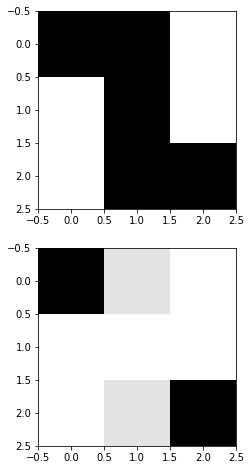Smooths the image (decreases the frequency) for example decreasing the difference from 5x to 2x.

#### 2.B.b

\begin{bmatrix} -1
0
1 \end{bmatrix}

r = 20
x = np.array([[r, r , r/5],
[r, r, r/5],
[r, r , r/5]
])
fig, ax = plt.subplots(nrows=2, ncols=1, figsize=(5, 8))
ax.imshow(x, cmap='gray')
x_conv = ndimage.convolve(x, [[-1, 0, 1]])
x_conv[x_conv > 255] = 255
x_conv[x_conv < 0] = 0
ax.imshow(x_conv, cmap='gray')
print(x)
print(x_conv)

[[ 20.  20.   4.]
[ 20.  20.   4.]
[ 20.  20.   4.]]
[[  0.  16.  16.]
[  0.  16.  16.]
[  0.  16.  16.]]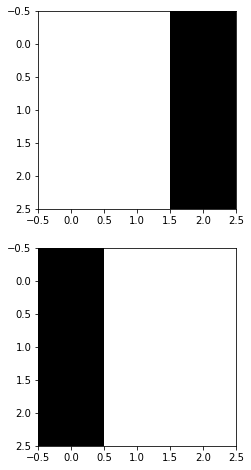Intensifies the difference between low and high value pixels

## 3 Calculate on Paper:

1. Norm: \begin{equation} x= \begin{bmatrix} 1+j
2
1-j \end{bmatrix} , y= \begin{bmatrix} 1
-1
1 \end{bmatrix} \end{equation
}

2. Inner Product of x and y. Orthonormal?

3. Find Degree: \begin{equation} x= \begin{bmatrix} 1
j
1-j \end{bmatrix} , y= \begin{bmatrix} -1
-j
j-1 \end{bmatrix} \end{equation
}

### 3.1 Norm

a = np.array([1+1j, 2, 1-1j]).reshape(-1, 1)
np.sqrt(np.vdot(a, a))

(2.8284271247461903+0j)

b = np.array([1, -1, 1]).reshape(-1, 1)
np.sqrt(np.vdot(b, b))

1.7320508075688772### 3.2 Inner Product

np.vdot(a, b)

0j


They are orthogonal not orthonormal.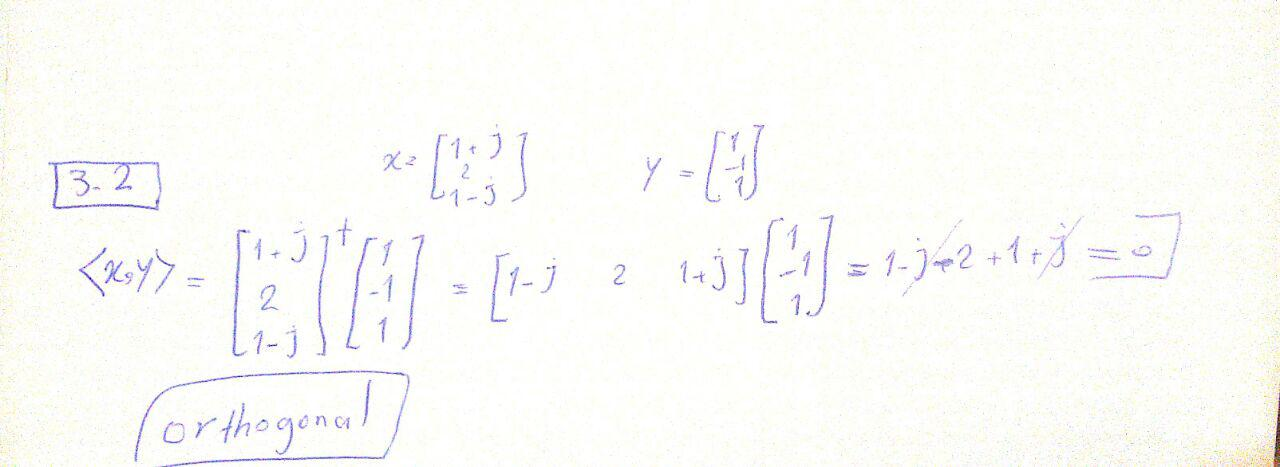### 3.3 Find Degree

x = np.array([1, 1j, 1-1j]).reshape(-1, 1)
y = np.array([-1, -1j, 1j-1]).reshape(-1, 1)

xy = np.vdot(x, y)
x_norm = np.sqrt(np.vdot(x, x))
y_norm = np.sqrt(np.vdot(y, y))
theta = np.arccos(xy.real/(x_norm.real* y_norm.real))
theta = theta * 180 / np.pi
theta

180.0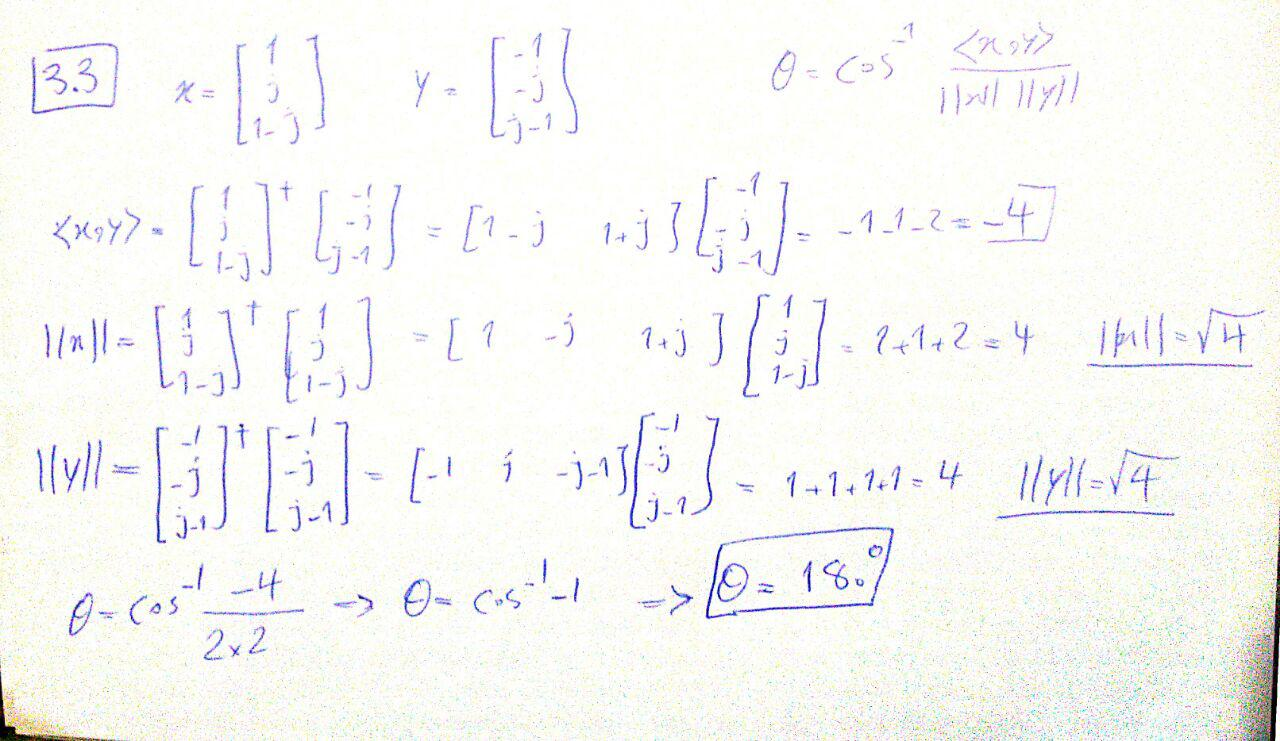## 4 Implementations

1. Implement make_gaussian(std, size)
2. Implement convolve2d(image, kernel)
3. Implement opencv_median_blur(image) from cv2
4. Implement opencv_gaussian_blur(image, std, size) from cv2
5. Analyze time and Results

### 4.1 Implement make_gaussian(std, size)

def gaussian(r2, std=1):
"""
Sample one instance from gaussian distribution regarding
given squared-distance:r2, standard-deviation:std and general-constant:k

:param r: squared distance from center of gaussian distribution
:param std: standard deviation

:return: A sampled number obtained from gaussian
"""
return np.exp(-r2/(2.*std**2)) / (2.*np.pi*std**2)

def make_gaussian(size=5, std=1):
"""
Creates a gaussian kernel regarding given size and std.
Note that to define interval with respect to the size,
I used linear space sampling which may has
lower accuracy from renowned libraries.

:param std: standard deviation value
:param size: size of the output kernel
:return: A gaussian kernel with size of (size*size)
"""
size = int(size) // 2
x, y = np.mgrid[-size:size+1, -size:size+1]
distance = x**2+ y**2
kernel = gaussian(r2=distance, std=std)
return kernel

print(make_gaussian(5, 1))


[[ 0.00291502  0.01306423  0.02153928  0.01306423  0.00291502]
[ 0.01306423  0.05854983  0.09653235  0.05854983  0.01306423]
[ 0.02153928  0.09653235  0.15915494  0.09653235  0.02153928]
[ 0.01306423  0.05854983  0.09653235  0.05854983  0.01306423]
[ 0.00291502  0.01306423  0.02153928  0.01306423  0.00291502]]


### 4.2 Implement convolve2d(image, kernel)

def convolve2d(image, kernel):
"""
Applies 2D convolution via provided kernel over given image

:param image: input image in grayscale mode
:param kernel: kernel of convolution
:return: A convolved image with 'same' size using zero-padding
"""
# you do not need to modify these, but feel free to implement however you know from scratch
kernel       = np.flipud(np.fliplr(kernel))  # Flip the kernel, if it's symmetric it does not matter
k = kernel.shape
padding      = (k - 1)
offset       = padding // 2
output       = np.zeros_like(image)
# Add zero padding to the input image
image_padded[offset:-offset, offset:-offset] = image

# implement the convolution inside the inner for loop
for y in range(image.shape):
for x in range(image.shape):
output[y,x] = np.sum(kernel * image_padded[y:y+k, x:x+k])
return output

x = np.random.randn(5, 5)
f = np.arange(9).reshape(3 ,3)
scipy_out = ndimage.convolve(x, f, mode='constant', cval=0)
our_out = convolve2d(x, f)

print('Results from scipy and out implementation are same? Answer:\n',scipy_out.astype('uint8') == our_out.astype('uint8'))

Results from scipy and out implementation are same? Answer:
[[ True  True  True  True  True]
[ True  True  True  True  True]
[ True  True  True  True  True]
[ True  True  True  True  True]
[ True  True  True  True  True]]


### 4.3 Implement opencv_median_blur(image, size) from cv2

In Open CV, size argument also exists and it is mandatory which you omitted.

import cv2

def opencv_median_blur(image, size):
"""
Applies median filter regarding given window size

:param image: open cv or numpy ndarray image
:param size: size of median kernel
:return: An open cv image
"""
return cv2.medianBlur(image, size)


### 4.4 Implement opencv_gaussian_blur(image, std, size) from cv2

def opencv_gaussian_blur(image, size, std):
"""
Applies gaussian filter to smooth image

:param image: Open cv or numpy ndarray image
:param size: The size of gaussian filter
:param std: if 0, it will be calculated automatically, otherwise for x and y should be indicated.
:return: An open cv image
"""
return cv2.GaussianBlur(src=image, ksize=(size, size), sigmaX=std, sigmaY=std)


### 4.5 Analyze time and Results

In Open CV, size argument also exists for median and it is mandatory which you omitted so I changed the for loop even though you said no to, to add size=11 to median.

The reason I chose 11 is that in gaussian you used 11 too.

You had a type: cv should be cv2 in codes
import os
import time

src_path = 'images_noisy/'
dst_path = 'images_filtered/'
names = os.listdir(src_path)

# Do not modify these for loops
for name in names:
src_name = src_path + name

# gaussian blur using your implementation
start = time.time()
kernel1 = make_gaussian(std=1, size=11)
blur1 = convolve2d(image, kernel1)
dt1 = time.time() - start
dst_name1 = dst_path + name + '.bmp'
cv2.imwrite(dst_name1, blur1)

# gaussian blur using opencv implementation
start = time.time()
blur2 = opencv_gaussian_blur(image, std=1, size=11)
dt2 = time.time() - start
dst_name2 = dst_path + name + '_CV.bmp'
cv2.imwrite(dst_name2, blur2)

# median blur
start = time.time()
blur3 = opencv_median_blur(image, 11)  ################# THIS CODE ONLY WORKS IF SIZE being provided.
dt3 = time.time() - start
dst_name3 = dst_path + name + '_median.bmp'
cv2.imwrite(dst_name3, blur3)
print('Our gaussian time:{}, opencv gaussian time:{}, opencv median time:{}'.format(dt1, dt2, dt3))

Our gaussian time:1.588090181350708, opencv gaussian time:0.0009992122650146484, opencv median time:0.004996776580810547
Our gaussian time:1.8719286918640137, opencv gaussian time:0.0, opencv median time:0.003996610641479492
Our gaussian time:2.5495409965515137, opencv gaussian time:0.0010004043579101562, opencv median time:0.009994029998779297
Our gaussian time:1.4371778964996338, opencv gaussian time:0.0009999275207519531, opencv median time:0.004997730255126953

##### Comparing Time Consumption

First of all, our implementation time is much bigger that open cv’s and it is completely obvious. If we want to compare gaussian, out convolution2d function has not been implemented efficiently which the main reason is the 2 for loops for iterating over image and computing convolution. The other reason is that opencv’s core is in C++ and we know that it is much faster (3x) even from efficient pure python code.

But if want to compare gaussian and median time, we can say that median operation is ordered and needs sorting every time and furthermore, it cannot be implemented using kernel logics which means most of the optimizations related to parallel computing or efficient vectorization will be lost, so that is why we can see an explosion about 10x increase in time.

Note: As you can see one of the times are 0.0 and that is because time module cannot handle very small numbers in small code snippets, so usually timeit module is preferred.

##### Comparing Quality
src_path = 'images_noisy/'
dst_path = 'images_filtered/'
names = os.listdir(src_path)

fig, ax = plt.subplots(nrows=4, ncols=4, figsize=(20, 15))
# Do not modify these for loops
for idx, name in enumerate(names):
src_name = src_path + name

# gaussian blur using your implementation
kernel1 = make_gaussian(std=1, size=11)
blur1 = convolve2d(image, kernel1)

# gaussian blur using opencv implementation
blur2 = opencv_gaussian_blur(image, std=1, size=25)

# median blur
blur3 = opencv_median_blur(image, 5)

# plotting
ax[idx, 0].set_title('Original')
ax[idx, 1].set_title('our_gaussian')
ax[idx, 2].set_title('cv gaussian')
ax[idx, 3].set_title('cv median')
ax[idx, 0].axis('off')
ax[idx, 1].axis('off')
ax[idx, 2].axis('off')
ax[idx, 3].axis('off')

ax[idx, 0].imshow(image, cmap='gray')
ax[idx, 1].imshow(blur1, cmap='gray')
ax[idx, 2].imshow(blur2, cmap='gray')
ax[idx, 3].imshow(blur3, cmap='gray')First two row of images correspond to the images with normal noise. So A gaussian blur can easily handle this kind of noises by reducing the frequency between pixels in the vicinity of each other without making image to blurry. But median, cannot find the good pixel to retain information without making the image completely blurry, because the noise is distributed almost between all values so median cannot do much and as you can see in the last column of the two first row, images are just blurry without removing noise.

But about the latter second rows, we can see the salt and pepper noise and this time, gaussian cannot do much because when it tries to decrease intensification, the output of convolution consists of 0 or 255 values and they will simply dominate other values so the output will be a blurry image without removing salt or peppers. They are still completely visible. But when we have such a noise in our images, we know they are minority in number so taking median, will focus on pixels that are more common in each window, so 0 or 255 values will not effect in median operation because they are almost rare in each window, so the output of images using this median filter will be blurry image without salt and peppers.